ISSN ONLINE(2319-8753)PRINT(2347-6710)

All submissions of the EM system will be redirected to Online Manuscript Submission System. Authors are requested to submit articles directly to Online Manuscript Submission System of respective journal.

# Alternate Iterative Algorithms for Minimization of Non-linear Functions

 K. Karthikeyan Associate Professor, Mathematics division, VIT University, Vellore, Tamil nadu, India Related article at Pubmed, Scholar Google

Visit for more related articles at International Journal of Innovative Research in Science, Engineering and Technology

## Abstract

Numerical Optimization algorithms presents the most effective methods in continuous optimization. It responds to the growing interest in optimization in engineering, science, and business by focusing on the methods that are best suited to practical problems. In this article, we propose some alternative iterative algorithms, with different order of convergence for minimization of non-linear functions. Then comparative study among the proposed algorithms and Newton’s algorithm is established by means of examples

### Keywords

Non-linear functions, Newton’s method, Ostrowski’s method, Eighth-order Convergence

### INTRODUCTION

An optimization problem consists of maximizing or minimizing a real function by systematically choosing input values from within an allowed set and computing the value of the function. The generalization of optimization theory and techniques to other formulations comprises a large area of applied mathematics. More generally, optimization includes finding "best available" values of some objective function given a defined domain, including a variety of different types of objective functions and different types of domains. Many optimization problems with or without constraints arise in various fields such as science, engineering, economics, management sciences, etc., where numerical information is processed. In recent times, many problems in business situations and engineering designs have been modeled as an optimization problem for taking optimal decisions. In fact, numerical optimization techniques have made deep in to almost all branches of engineering and mathematics. Several methods [8, 10, 16, 20, 21] are available for solving unconstrained minimization problems. These methods can be classified in to two categories as non gradient and gradient methods. The non gradient methods require only the objective function values but not the derivatives of the function in finding minimum. The gradient methods require, in addition to the function values, the first and in some cases the second derivatives of the objective function. Since more information about the function being minimized is used through the use of derivatives, gradient methods are generally more efficient than non gradient methods. All the unconstrained minimization methods are iterative in nature and hence they start from an initial trial solution and proceed towards the minimum point in a sequential manner. To solve unconstrained nonlinear minimization problems arising in the diversified field of engineering and technology, we have several methods to get solutions. For instance, multi- step nonlinear conjugate gradient methods , a scaled nonlinear conjugate gradient algorithm, a method called, ABS-MPVT algorithm  are used for solving unconstrained optimization problems. Newton’s method  is used for various classes of optimization problems, such as unconstrained minimization problems, equality constrained minimization problems. A proximal bundle method with inexact data  is used for minimizing unconstrained non smooth convex function. Implicit and adaptive inverse preconditioned gradient method  is used for solving nonlinear minimization problems. A new algorithm  is used for solving unconstrained optimization problem with the form of sum of squares minimization. A derivative based algorithm  is used for a particular class of mixed variable optimization problems. A globally derivative – free decent method  is used for nonlinear complementarity’s problems.
Vinay Kanwar et al.  introduced new algorithms called, external touch technique and orthogonal intersection technique for solving the non linear equations. A.M.Ostrowski’s introduced fourth order convergence iteration scheme for solving non linear equations. Sharma and Guha introduced a family of modified Ostrowski’s methods with accelerated sixth order convergence. Chun and Ham  proposed some sixth order variants of Ostrowski’s root finding methods. Kou. et al  introduced some variants of Ostrowski’s method with seventh order convergence. Grau et.al proposed an improvement to Ostrowski’s root finding method. Miquel Grau-Sanchez proposed improvements of the efficiency of some three step iterative like Newton’s methods. Recently, Jisheng Kou and Xiuhua Wang [ 20] introduced some improvements of Ostrowski’s method with order of convergence eight. In this article, we introduce alternative algorithms for minimization of non linear functions and comparative study is established among the new seven algorithms with Newton’s algorithm by means of examples.

### NEW ALGORITHMS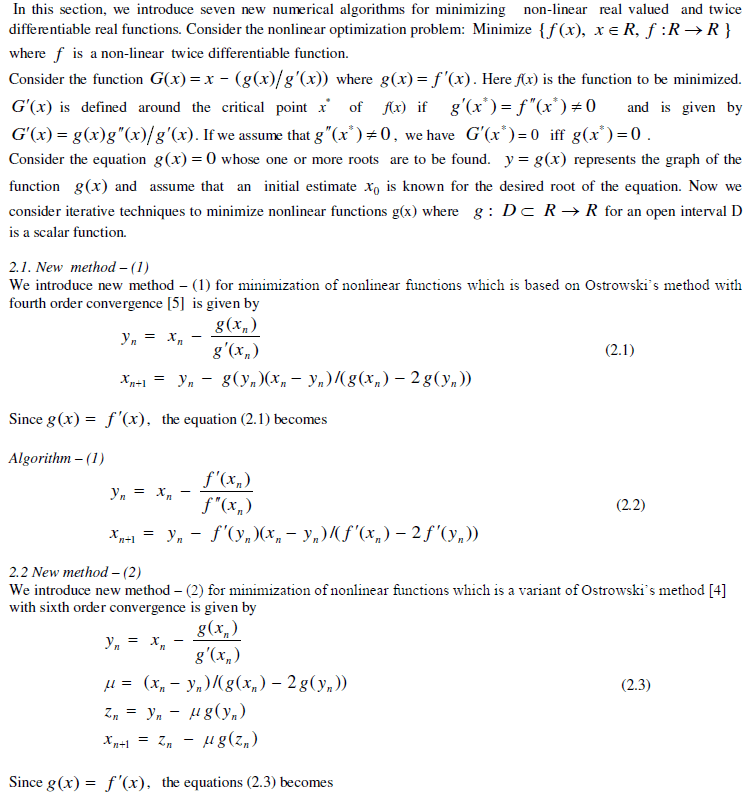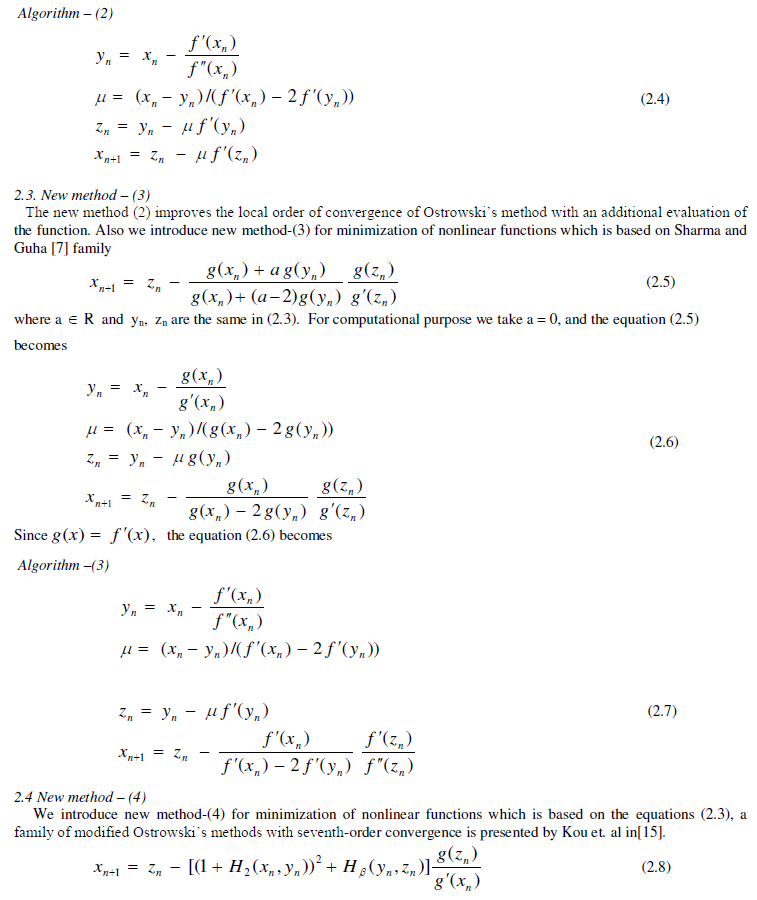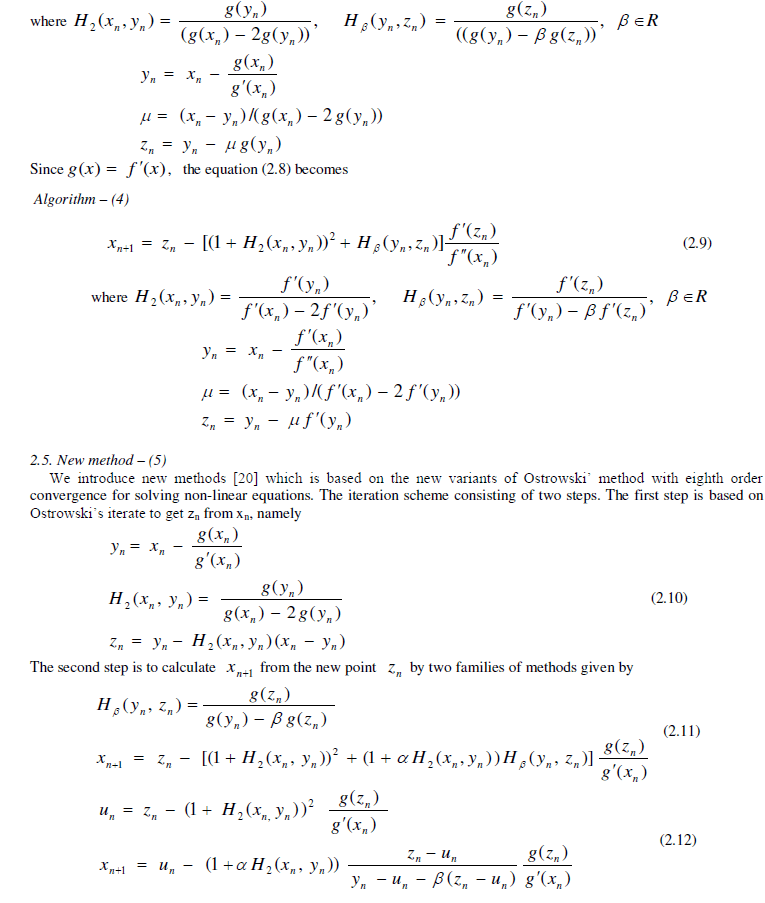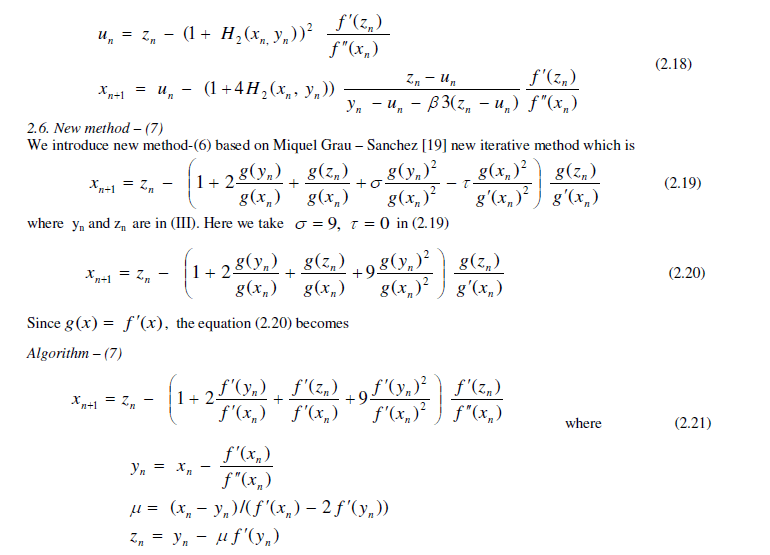### NUMERICAL ILLUSTRATIONS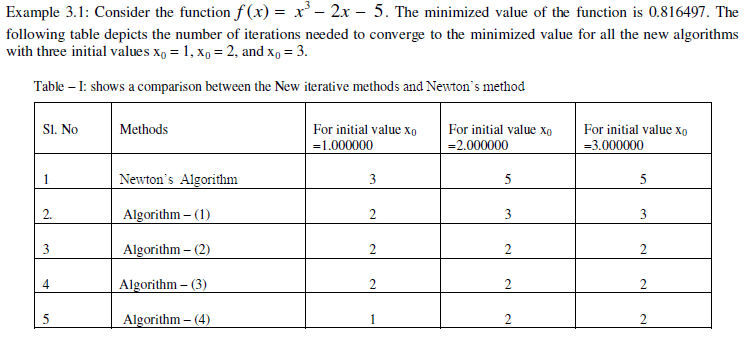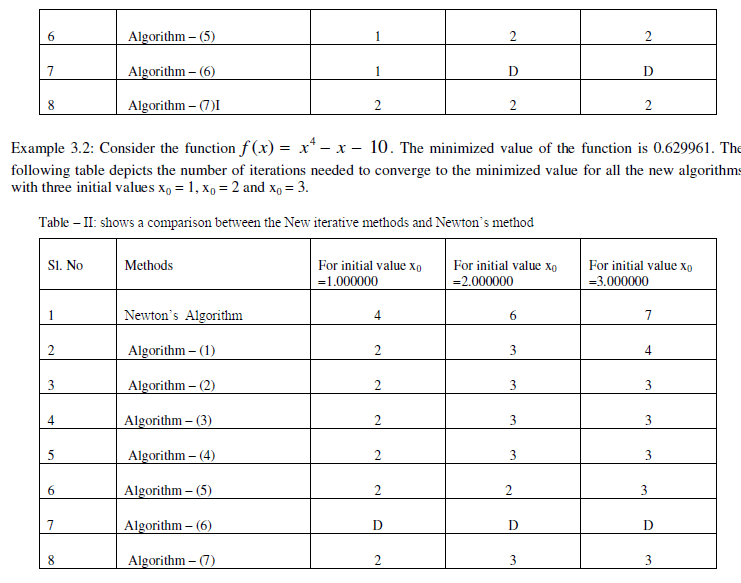### CONCLUSION

In this paper, we introduced seven alternative numerical algorithms for minimization of nonlinear unconstrained optimization problems and compared with Newton’s method. It is clear from the above numerical results that the rate of convergence of algorithm (1) to algorithm(7) are in general faster than Newton’s algorithm. In particular algorithm(5) and algorithm (4) converge much better than the remaining algorithms. In real life problems, the variables can not be chosen arbitrarily rather they have to satisfy certain specified conditions called constraints. Such problems are known as constrained optimization problems. In near future, we have a plan to extend the proposed new algorithms to constrained optimization problems.

### References

. Andrei, N, “ A scaled nonlinear conjugate gradient algorithm for unconstrained minimization”, Optimization Vol. 57(4), pp.549 – 570, 2008.

. Chehab,J.-P and Raydon, M, “Implicit and adaptive inverse preconditioned gradient methods for nonlinear problems ”, Applied Numerical Mathematics, Vol.55(1), pp.32 -47, 20005.

. Ford.J.A., Narushima, Y. and Yabe,H, “ Multi-step nonlinear conjugate gradient methods for constrained minimization”, Computational Optimization and application Vol.40(2), pp.191 -216, 2008.

. Grau. M. and Diaz-Barrero. J.L, “ An improvement to Ostrowski root finding method”, Appl. Math. Comput., Vol.173, pp.450 – 456, 2006.

. Ostrowski.A.M, “Solutions of equations and Systems of Equations”, Academic Press, New York, 1960.

. Hu,Y., Su, H. and Chu, J., “A new algorithm for unconstrained Optimization Problem with the form of sum of squares minimization”, Conference proceedings - IEEE international conference on systems, man and cybernetics Vol. 7, pp..6108 – 6112, 2004.

. Sharma.J.R. and Guha.R.K., “ A family of modified Ostroswski methods with accelerated sixth order convergence ”, Appl. Math. Comput. Vol.190, pp.111– 115, 2007.

. Kanniappan, P. and Thangavel, K, " Unidimensional Search Scheme Using Identric Mean for Optimization Problems ", Opsearch, Vol.38 (2), pp.197-209, 2001.

. Lucid, S. and Piccialli,V, ” A derivative based algorithm for a particular class of mixed variable optimization problems ”, Optimization Methods and Software, Vol.19(3-4), pp.371-387, 2004.

.Mohan C Joshi and Kannan M Moudgalya, " Optimization theory and Practice ", Narosa Publication House, New Delhi, 2004.

. Chun.C and Ham.Y, “Some sixth order variants of Ostrowski’s root finding methods ”, Appl. Math. Comput., Vol.193, pp.389 – 394, 2007.

. Pang, L.P., Spedicato, E., Xia, Z.Q. and Wang, W, “A method for solving the system of linear equations and linear inequalities ”, Mathematical and Computer Modelling, Vol. 46(5-6), pp. 823 – 836, 2007

. Polyak, B.T, “Newton’s method and its use in optimization”, European Journal of Operational research Vol.181(3), pp.1086 – 1096, 2007.

. Qi, H,-D, “A globally derivative-free descent method for nonlinear complementarity problems”, Journal of Computational Mathematics Vol.18(3), pp.251-264, 2000.

. Kou.J., Li.Y., Wang. X, “Some variants of Ostrowski’s method with seventh order convergence”, J. Comput. Appl. Math., 209, pp.153 – 159, 2007.

. Reklaitis, G.V., Ravindran, A. and Ragsdell, K.M., " Engineering optimization methods and applications " , John Wiley and sons, New York, 1983.

. Shen, J., Xia, Z.-Q. and Pang, L.P, “A proximal bundle method with inexact data for convex non differentiable minimization ”, Nonlinear Analysis, Theory, Methods and Applications, Vol. 66(9), pp. 2016 -2027, 2007.

. Vinay Kanwar, Sukhjit Singh, Sharma, J.R. and Mamta, " New numerical techniques for solving non-linear equations ", Indian Journal of Pure and Applied Mathematics, Vol. 34(9), pp.1339 – 1349, 2003.

. Miquel Grau-Sanchez, “Improvements of the efficiency of some three step iterative like Newton’s method”, Numer. Math. Vol.107, pp.131 – 146, 2007.

. Jisheng Kou and Xiuhua Wang, “Some improvements of Ostrowski’s method”, Applied Mathematics letters, Vol. 23, pp. 92 – 96, 2010.

. Karthikeyan.K, “ New efficient algorithms for minimization of non linear functions”, International Journal of Pure and Applied Mathematics, Vol. 86(5), pp.811-818, 2013.

. Karthikeyan.K, “Efficient algorithms for minimization of non linear functions”, Applied Mathematical Sciences , Vol. 4(69), pp. 3437-3446, 2010.